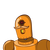# the denominator of fraction is 2 more than numerator. if 1 is subtracted from numerator and 1is added to denominator the fraction

the denominator of fraction is 2 more than numerator. if 1 is subtracted from numerator and 1is added to denominator the fraction becomes 1/2 what is that fraction.​

### 1 thought on “the denominator of fraction is 2 more than numerator. if 1 is subtracted from numerator and 1is added to denominator the fraction”

1.5/7

Step-by-step explanation:

let the numerator be x

the denominator is 2more so,

x/x+2

if 1added to the denominator and 1subtracted from the numerator,

x-1/x+2+1 =

x-1/x+3 =1/2

cross multiply

2(x-1)= x+3

2x-2 = x+3

2x- x = 3+2

x= 5

so the numerator is 5 and so denominator would be x+2 i.e 5+2 = 7

ans is 5/7